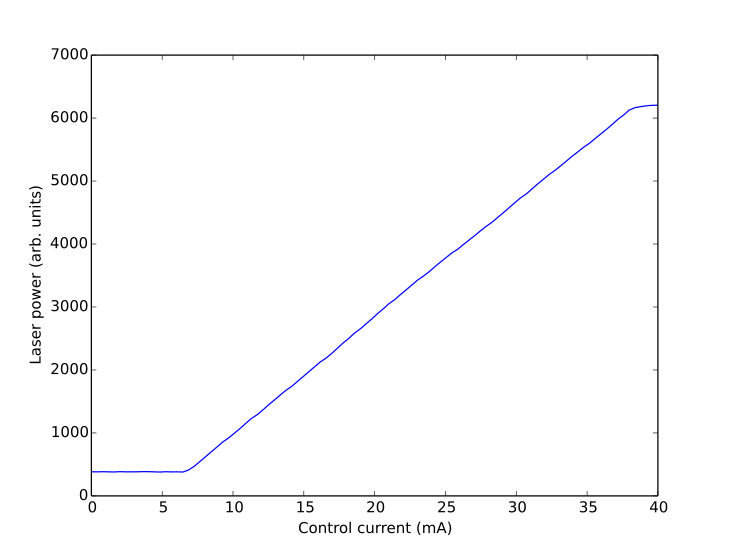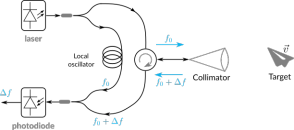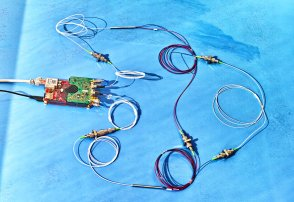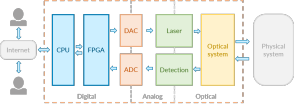# Control the laser board with Python

2016-01-07

Koheron laser board can be controlled remotely through the network via TCP connection. In this post, we show how to control the board using the provided Python API. Before doing anything interesting, we need to connect to the board.

## Connect to the board

In what follows, we connect the board to a router using an Ethernet cable and plug the power supply. After a few seconds, the router has assigned an IP address to the board.In our local network, all connected devices have an IP address of the form 192.168.1.X. The number X, which is between 0 and 255, is displayed in binary form on the 8 yellow LEDs of the board. In our case, only LED2 and LED3 are lit so X = 2^2 + 2^3 = 12. The board IP address is thus 192.168.1.12.

## My first Python script

The Python API is available on GitHub. Let's first connect to the board using the IP address found above:

# Import the required packages
from koheron import connect
from drivers import Laser

# Connect to the board
host = '192.168.1.12'
client = connect(host, instrument='oscillo')

# Initialize the driver
laser = Laser(client)


We can now use the laser object to start the laser and drive it with a current of 30 mA:

current = 30 #mA
laser.start()
laser.set_current(current)


Laser current and laser power can be monitored from the Python API:

# Monitor laser current
print('Measured laser current = {:.2f} mA'.format(laser.get_measured_current()))

# Monitor laser power
print('Measured laser power = {:.2f} Arb. Units'.format(laser.get_measured_power()))


## Laser power vs. current

As an exercise, one can imagine a little more complex script to generate the figure below:The laser does not output any power until a current of about 6 mA. Above this threshold, laser power increases linearly with the current. The saturation can be explained by the laser current protection which prevents the laser to be driven above 37 mA. Below is the script that has been used to produce the figure (also available at this link):

#!/usr/bin/env python
# -*- coding: utf-8 -*-

import os
import time
import numpy as np
import matplotlib.pyplot as plt

from koheron import connect
from drivers import Laser

host = os.getenv('HOST','192.168.1.100')
client = connect(host, instrument='oscillo')
laser = Laser(client)

laser.start()
laser.set_current(0)
time.sleep(0.1)

current_max = 40
currents = np.linspace(0,current_max, num=100)

laser_powers = 0 * currents
measured_currents = 0 * currents

for i, current in enumerate(currents):
laser.set_current(current)
time.sleep(0.02)
laser_powers[i] = laser.get_measured_power()
measured_currents[i] = (0.0001/21)*(laser.get_measured_current())
print('laser power = ' + str(laser_powers[i]) + ' arb. units')

# Plot
fig1 = plt.figure('Power vs current')
plt.plot(currents, laser_powers)
plt.xlabel('Control current (mA)')
plt.ylabel('Laser power (arb. units)')

np.savetxt('power_vs_current.csv',
np.transpose([currents, laser_powers]),
delimiter=',',
fmt='%1.4e')

laser.stop()
plt.show()


## Source code

https://github.com/koheron/laser-development-kit

## Also tagged instrumentation### Build a Doppler lidar velocimeter### Web based interfaces for instrument control### A simple coherent laser sensor